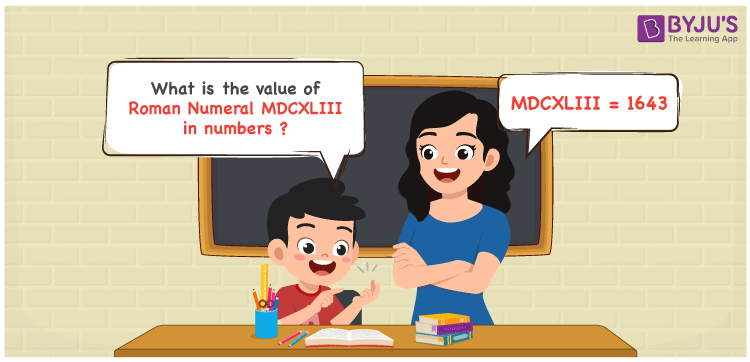Checkout JEE MAINS 2022 Question Paper Analysis : Checkout JEE MAINS 2022 Question Paper Analysis :

# MDCXLIII Roman Numerals

MDCXLIII Roman Numerals is 1643. The steps of conversion to transform the roman numerals in numbers can be grasped by the students in this article. As roman numerals is one of the important concepts in Mathematics, students have to focus on it and learn it from the basics. Therefore, the roman numerals MDCXLIII is written as 1643 in numbers.

 Number Roman Numeral 1643 MDCXLIII## How to Write MDCXLIII Roman Numerals in Numbers?

This section shows the steps used to indicate the given roman numerals MDCXLIII as 1643 in numbers.

MDCXLIII = M + D + C + (L – X) + I + I + I

MDCXLIII = 1000 + 500 + 100 + (50 – 10) + 1 + 1 + 1

MDCXLIII = 1643

## Video Lesson on Roman Numerals## Frequently Asked Questions on MDCXLIII Roman Numerals

### Why is MDCXLIII the roman numerals of 1643?

We know that

M is the roman numerals of 1000

DC is the roman numerals of 600

XL is the roman numerals of 40

III is the roman numerals of 3

So MDCXLIII is the roman numeral of 1643.

### Determine 1700 – 57.

We know that

1700 – 57 = 1643

Hence, 1700 – 57 is 1643 which is written as MDCXLIII.

### Find the remainder if MDCXLIII is divided by XII.

We know that

MDCXLIII = 1643

XII = 12

The remainder if MDCXLIII is divided by XII is XI.